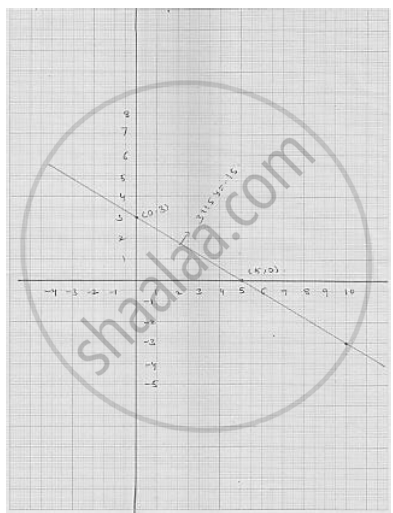# Draw the Graph of the Following Linear Equation in Two Variable : 3x + 5y = 15 - Mathematics

Draw the graph of the following linear equation in two variable : 3x + 5y = 15

#### Solution

We have
3 x + 5 y = 15
3x = 15 -5y
x = (15 - 5y)/ 3

Putting  y = 0, we get  x = (15 - 5 xx0)/ 3 = 5

Putting  y  =  3  we get x =  (15 - 5 xx3)/ 3 = 0

Thus, we get the following table giving the two points on the line represented by the
equation   3 x + 5 y - 15
Graph for the equation   3 x + 5 y - 15Concept: Graph of a Linear Equation in Two Variables
Is there an error in this question or solution?

#### APPEARS IN

RD Sharma Mathematics for Class 9
Chapter 7 Linear Equations in Two Variables
Exercise 7.3 | Q 1.5

Share